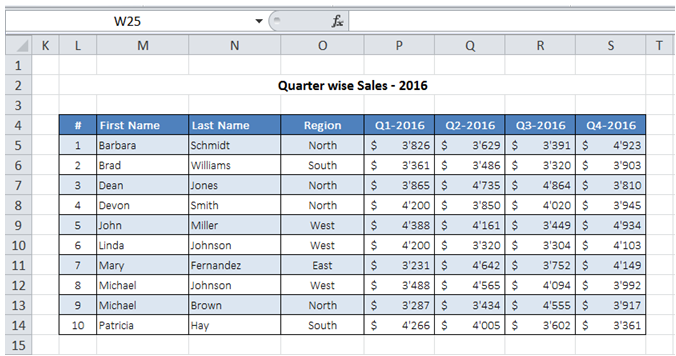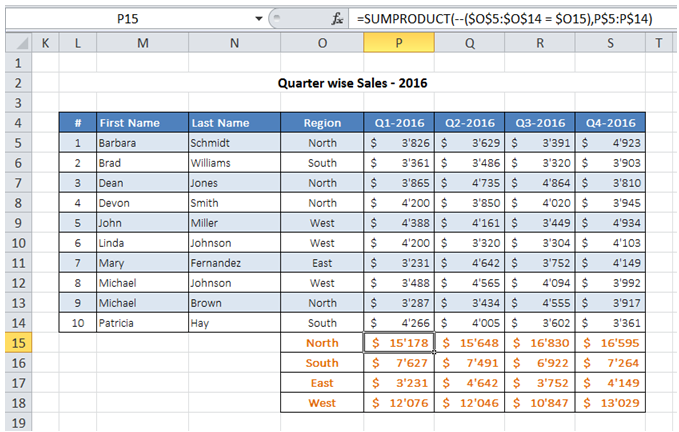## Summarize raw data with sumproduct in Excel

For example, I have my raw Sales Data information as shown below. In Excel, how can I get region wise Sales data?To do it in Excel, here is the answer:

a) Enter the formula =SUMPRODUCT(--(\$O\$5:\$O\$14 = \$O15),P\$5:P\$14) to get Q1-2016 Sales data for North Region.

The first argument "(\$O\$5:\$O\$14 = \$O15)" looks for all values in range O5 : O14 that are same as value specified in cell O15. The "--" converts TRUE/FALSE to 1 or 0.

In the first argument, notice it is mentioned as \$O15 so that the formula could be copied across rows.

The second argument picks and sums up the data if the first argument is TRUE for a value. Notice that the column reference P does not have a preceding \$ so that the formula could be copied across columns.

Drag the formula to get Sales data for the rest of the quarters / regions as shown below.